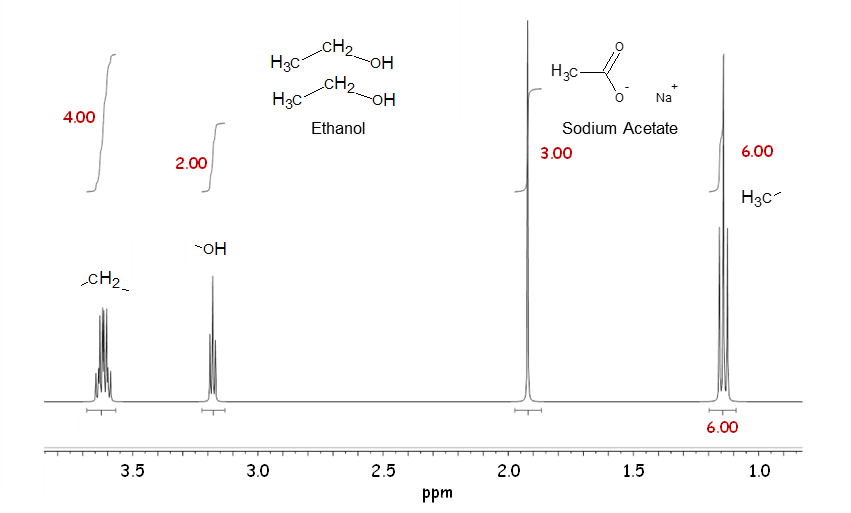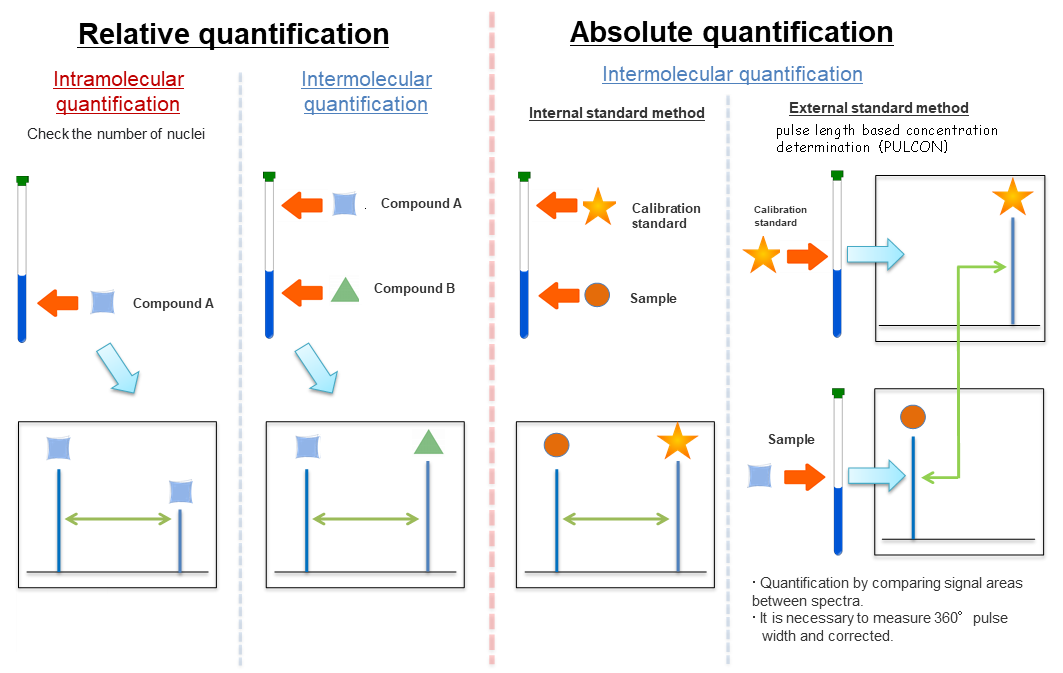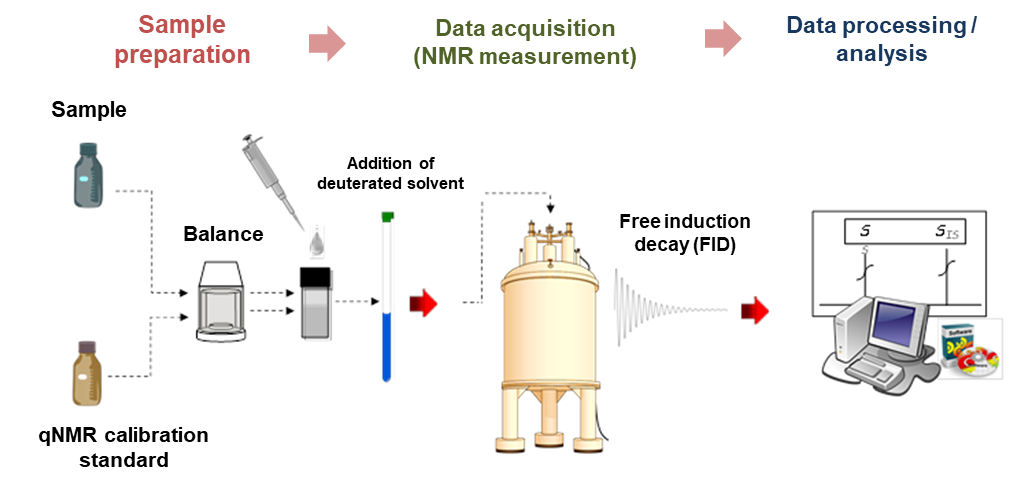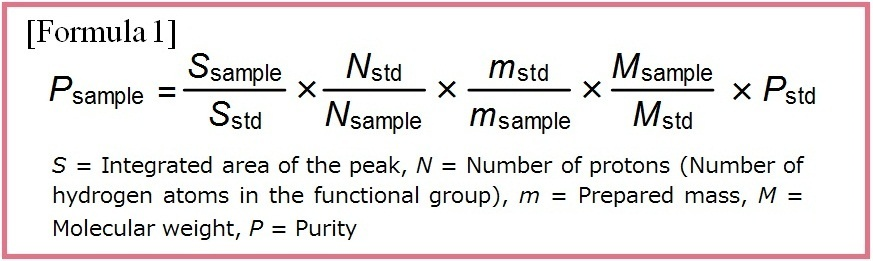# Quantitative NMR (qNMR)

NMR (Nuclear Magnetic Resonance) has been broadly used as a standard analysis method for structural determination of an organic compound. In NMR analysis, the ratio of the number of atomic nuclei in a compound corresponds to the ratio of the areas of the peaks in the spectrum. Therefore, the purity of the compound can be determined by performing a quantitative analysis.
In a purity determination using 1H NMR, a sample and a standard (an internal standard) having a known purity are mixed together and dissolved in a deuterated solvent. A quantitative value of the sample's purity can be calculated from the relationship among the areas of the spectral peaks originated from the sample and the standard, the number of protons, the prepared masses, and the molecular weights of the sample and the standard.

In quantitative analysis using 1H NMR (quantitative NMR; qNMR), the areas of the peaks of hydrogen atoms observed in the spectrum can be quantitatively compared. Therefore, determination of the purity and quantity of many compounds containing a hydrogen atom is possible with one standard as long as the signals of the sample and the standard do not overlap each other. Highly reliable purity and quantity measurement based on the amount of substance (moles) is possible using a standard certified to be traceable to the International System of Units (SI).

## Product Line-up

### What is qNMR?In the mixture solution of ethanol and sodium acetate in a molar ratio of 2: 1 (illustrated above), the ratio of the signal area is 6: 4: 3: 2. That is, the molar ratio of each component in the mixture solution can be determined by a simple ratio of normalized values, which are calculated by dividing the signal area by the number of nuclei. In the example above, the signal of methyl group of sodium acetate has a signal area of 3 and the number of nuclei of 3, so 3 dividing by 3 gives a normalized signal area of 1. Similarly, the signal area of the methyl, methylene, and hydroxy groups of ethanol are 6, 4, and 2, and when normalized, they are all 2. Therefore, it can be seen that the molar ratio of ethanol to sodium acetate is 2: 1. This simple example can be extended to much more complicated mixture solutions. Importantly, the calibration standard used in qNMR measurement does not have to be identical (the same type of substance) to the analyte to be measured. Therefore, with qNMR measurements, theoretically, it is possible to quantify all analytes using one type of calibration standard. This important property makes qNMR a versatile technique that can be applied to many fields and compounds.

### Outline of the Internal Standard MethodAs mentioned above, NMR performs intra and intermolecular quantification, and the types can be classified into relative and absolute quantification. Relative quantification is used for verification of the chemical structure by confirming the number of nuclei of each functional group in the molecule, and confirmation of the mixture ratio. On the other hand, absolute quantification is used for measuring purity and concentration in the field of drug analysis and quality assurance. The above is the outline of the internal standard method in the absolute quantification, which is gradually beginning to be more widely used triggered by its application to the official analytical method, such as Japanese Pharmacopeia.

### qNMR Measurement ProcedureqNMR procedures can be broadly divided into three parts (sample preparation, data acquisition (NMR measurement), and data processing) regardless of their type 1). Factors that cause bias or variability in the analytical results are potentially included in each part. However accurate analytical results can be obtained by using procedures suitable for qNMR.1. Miura T. et al. : Pham. Bull., 68(9), 868 (2020).

877-714-1920